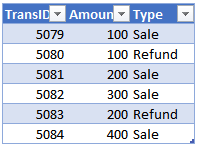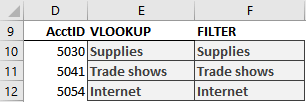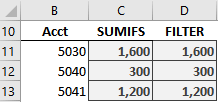# FILTER Function

Question: What is the best Excel function? Typical responses are VLOOKUP, INDEX, MATCH, XLOOKUP and SUMIFS, which are among Excel’s highlights.

Follow-up question: If there was a function that could do the work of these, would it be the Greatest of All Time (GOAT)?

Well, the FILTER function is too new to declare it the GOAT, but it certainly has the potential to be one of the greatest Excel functions ever.

## Details

The FILTER function can replace traditional lookup functions in many, but not all, situations. Even though FILTER may not be able to replace the others in every possible scenario, it does provide an alternative in many common scenarios.

One benefit of using FILTER instead of the assortment of others is simplicity. We only need to use one function and its arguments rather than several functions and their various arguments.

Another benefit is flexibility. Once we understand how FILTER retrieves one or more values, we’ll see how we can display them in cells or aggregate them with a helper function.

Plus, it supports multiple conditions (lookup values) and supports both AND and OR logic. But I’m getting ahead of myself. Let’s start by understanding the purpose and arguments of FILTER.

Note: At the time of this writing the FILTER function is available in Excel 365 only, and not in perpetual licenses such as Excel 2019, 2016, 2013, 2010 and so on.

## FILTER

The FILTER function is designed to return a subset of values from a range. The syntax is:

`=FILTER(array, include, [if_empty])`

Where:

• array is the range that has the values you wish to return;
• include is an expression that defines the condition(s); and
• [if_empty] optionally returns a specific value if nothing is found.

Let’s say we have exported a bunch of transactions and stored them in a table named Table1 (see Figure 1).Figure 1

We’d like to write a formula that retrieves the “Refund” transactions into another range in the workbook. We could accomplish this with:

`=FILTER(Table1, Table1[Type]=“Refund”)`

When we hit Enter, the formula returns the results (see Figure 2).Figure 2

The FILTER function retrieves all columns in Table1, but only includes the rows where the “Type” column value is equal to “Refund.” Since the formula returns multiple results, they spill out of the formula cell into the adjacent cells as needed.

As you can see, the FILTER function returns a subset of values from a range. In this example, the function returned multiple values from multiple rows and multiple columns. However, the function can also return a single column of values, a single row of values or a single value.

Since the function can return a single value, it can replace traditional lookup functions such as VLOOKUP. Since the function is able to return multiple values, we can aggregate the results with a helper function like SUM to replace conditional summing functions such as SUMIFS. But we are not limited to SUM; we can use a variety of helper functions to combine results, such as COUNT, AVERAGE, MIN, MAX, TEXTJOIN, ARRAYTOTEXT and so on.

It’s time to dig into some additional illustrations to understand how we can use FILTER as an alternative to traditional lookup functions. We’ll start with the venerable VLOOKUP.

### VLOOKUP

VLOOKUP is often used to retrieve a related value. For example, we need to find an account number and return the related account name. Imagine our chart of accounts is stored in Table2 (see Figure 3).Figure 3

We need to retrieve the account names for our exported transactions. Assuming we wanted to search for an account id stored in D10, we could retrieve the related account name with either VLOOKUP or FILTER:

```=VLOOKUP(D10, Table2, 2, 0)
=FILTER(Table2[AcctName],Table2[AcctID]=D10)```

Fill both formulas down and they provide the same results (see Figure 4).Figure 4

In this scenario, FILTER can replace VLOOKUP. Now let’s change the scenario a bit.

Column order is important to VLOOKUP. It assumes the lookup column (eg, AcctID) is the first column in the lookup range (Table2). But what if the column order was switched around so that AcctName was first? In this situation, Excel users typically move away from VLOOKUP and use INDEX/MATCH instead. So, let’s see how FILTER compares to INDEX/MATCH.

### INDEX/MATCH

INDEX/MATCH is often used as an alternative to VLOOKUP because the column order doesn’t matter. For example, consider the lookup table (see Figure 5).Figure 5

The lookup column (AcctID) is not the first column in the lookup range. It lies to the right of the return column (AcctName). Rather than manually switch the column order and use VLOOKUP, Excel users will often use INDEX/MATCH.

Assuming we are trying to find the account ID stored in D10, we can retrieve the corresponding account name with either INDEX/MATCH or FILTER:

```=INDEX(Table3[AcctName], MATCH(D10,Table3[AcctID], 0))
=FILTER(Table3[AcctName],Table3[AcctID]=D10)```

Fill both formulas down and they provide the results (see Figure 6).Figure 6

In these examples, we’ve retrieved a single column value, the account name. But what happens if we want to return values from multiple columns? Rather than write separate formulas for each, an Excel user can use XLOOKUP. So, let’s look at that next.

### XLOOKUP

XLOOKUP can return multiple column values.

Consider the data range in Figure 7.Rather than return a single column value, such as “Jan,” we want to write one formula that retrieves “Jan,” “Feb,” and “Mar” values. We can accomplish this with XLOOKUP and FILTER. Assuming our lookup value is in B12, both formulas retrieve “Jan,” “Feb,” and “Mar” values:

```=XLOOKUP(B12, Table4[Dept],Table4[[Jan]:[Mar]])
=FILTER(Table4[[Jan]:[Mar]],Table4[Dept]=B12)```

Fill both formulas down and they return the same results (see Figure 8).Figure 8

In this example, we retrieved values from multiple columns. But what if we had a situation where we had multiple matching rows and wanted to return the sum of them? Well, VLOOKUP, INDEX/MATCH and XLOOKUP are designed to stop at the first matching row. So, in this situation, an Excel user would turn to a conditional summing function such as SUMIFS.

### SUMIFS

SUMIFS returns the sum of all matching rows. Let’s say we have a table full of transactions (see Figure 9).Figure 9

If we wanted to return the sum of all account 5030 transactions, we would need to move away from traditional lookup functions because they stop at the first matching row. Instead, we could use SUMIFS to compute the sum of all 5030 transactions and return the total of 1,600. If we tried this with FILTER, we wouldn’t
get the total; we would get the individual cell values, such as 300 and 1,300. The good news is that it’s easy to combine these values by wrapping the SUM function around the FILTER function.

Assuming the account is stored in B11, both formulas return the sum of the matching transactions:

```=SUMIFS(Table5[Amount], Table5[Acct],B11)
=SUM(FILTER(Table5[Amount],Table5[Acct]=B11))```

We fill both formulas down and confirm they return the same results (see Figure 10).Figure 10

Wow! Once we understand that we can wrap an aggregate function around the results of a FILTER function, it opens many other interesting applications. For example, we could use COUNT, AVERAGE, MIN, MAX and so on to aggregate numeric values. If FILTER returned a bunch of text values, we could combine them using functions such as TEXTJOIN, ARRAYTOTEXT or CONCAT.

Additionally, unlike VLOOKUP, FILTER supports multiple conditions (lookup values) and unlike SUMIFS, FILTER supports both AND and OR logic when processing multiple conditions.

As you can see, the FILTER function provides an interesting alternative to the variety of functions Excel users typically use to accomplish such tasks. And remember, Excel rules!

Posted in### Jeff Lenning

I love sharing the things I've learned about Excel, and I built Excel University to help me do that. My motto is: Learn Excel. Work Faster.

### Excel is not what it used to be.

You need the Excel Proficiency Roadmap now. Includes 6 steps for a successful journey, 3 things to avoid, and weekly Excel tips.